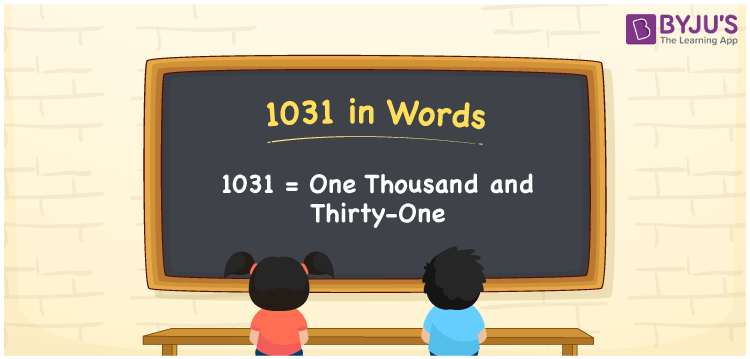# 1031 in words

1031 in words is written as One Thousand and Thirty One. 1031 represents the count or value. The article on Counting Numbers can give you an idea about count or counting. The number 1031 is used in expressions that relate to money, distance, length, year and others. Let us consider an example for 1031. ”The year One Thousand and Thirty One was a non-leap year (regular year).”

 1031 in words One Thousand and Thirty One One Thousand and Thirty One in Numbers 1031

## 1031 in English Words## How to Write 1031 in Words?

We can convert 1031 to words using a place value chart. The number 1031 has 4 digits, so let’s make a chart that shows the place value up to 4 digits.

 Thousands Hundreds Tens Ones 1 0 3 1

Thus, we can write the expanded form as:

1 × Thousand + 0 × Hundred + 3 × Ten + 1 × One

= 1 × 1000 + 0 × 100 + 3 × 10 + 1 × 1

= 1031

= One Thousand and Thirty One.

1031 is the natural number that is succeeded by 1030 and preceded by 1032.

1031 in words – One Thousand and Thirty One.

Is 1031 an odd number? – Yes.

Is 1031 an even number? – No.

Is 1031 a perfect square number? – No.

Is 1031 a perfect cube number? – No.

Is 1031 a prime number? – Yes.

Is 1031 a composite number? – No.

## Solved Example

1. Write the number 1031 in expanded form

Solution: 1 × 1000 + 0 × 100 + 3 × 10 + 1 × 1

We can write 1031 = 1000 +000 +30 + 1

= 1 × 1000 + 0 × 100 + 3 × 10 + 1 × 1

## Frequently Asked Questions on 1031 in words

Q1

### How to write 1031 in words?

1031 in words is written as One Thousand and Thirty One.
Q2

### Is 1031 a perfect square number?

No. 1031 is not a perfect square number.
Q3

### Is 1031 a prime number?

Yes. 1031 is a prime number.